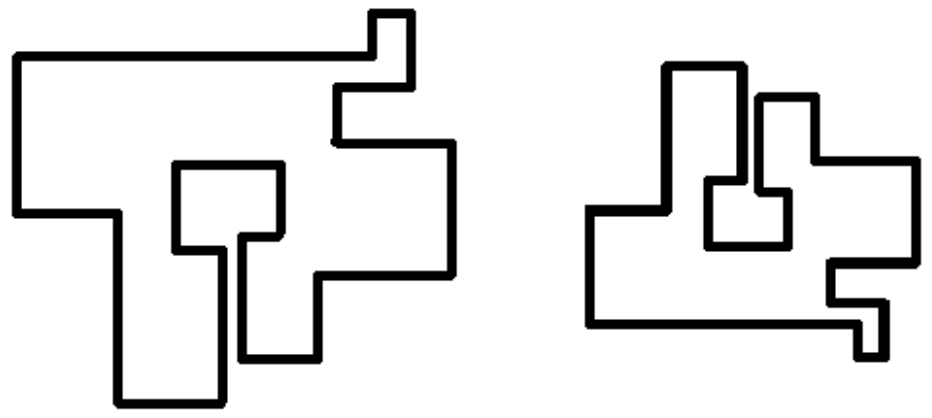시간 제한 메모리 제한 제출 정답 맞은 사람 정답 비율
2 초 512 MB 0 0 0 0.000%

## 문제Two polygons are similar if you can obtain the second polygon by a combination of scaling, rotating and flipping operations on the first polygon.

You are given two polygons whose sides are all horizontal and vertical lines. You are to determine if the two polygons are similar.

## 입력

The input contains one or more test cases. Each case contains two polygon definitions. A polygon definition is the number of the vertices of the polygon in the first line, followed by an ordered pair (x, y) of the vertices for the following lines. Format for each test case is shown below:

<number of vertices (n) of polygon 1>
<x1,y1>
<x2,y2>
.
.
.
<xn,yn>
<number of vertices (m) of polygon 2>
<x1,y1>
<x2,y2>
.
.
.
<xm,ym>

Input is terminated by “0”.

You can assume that the polygon resulting from connecting the vertices in sequence has only horizontal and vertical lines, and that the polygon formed is simple (i.e. it has no intersecting or overlapping edges). All vertex coordinates are integers, ranging from -30000 to +30000.

## 출력

Output YES if the polygons are similar; NO, if otherwise – one line per test case.

## 예제 입력 1

6
0,0
0,2
1,2
1,1
2,1
2,0
6
0,0
0,2
-1,2
-1,1
-2,1
-2,0
4
0,0
1,0
1,1
0,1
4
0,0
2,0
2,1
0,1
0


## 예제 출력 1

YES
NO